﻿ 基于分数阶PI<sup><i>λ</i></sup>D<sup><i>μ</i></sup>的船舶航向控制
 舰船科学技术2016, Vol. 38Issue (5): 87-90PDF

1. 海军驻大连426厂军事代表室, 辽宁 大连 116000 ;
2. 东部战区海军装备部信息系统处, 浙江 舟山 316000 ;
3. 中国船舶重工集团公司第七〇七研究所 九江分部, 江西 九江 332007

Marine course control based on fractional-order PIλDμ
ZHANG An-ming1, SUN Jie2, HUANG Jing3, LIU Jian3
1. The Navy Representative Office in No. 426 Shipyard, Dalian 116000, China ;
2. Eastern Theater Naval Armaments Department Information Systems Branch, Zhoushan 316000, China ;
3. Jiujiang Branch of the 707 Research Institute of CSIC, Jiujiang 332007, China
Abstract: The sailing marine vehicles are disturbed by the wind, wave and current on the ocean, which cause the model parameters' uncertainty for ship motion in different sailing velocity. In this paper, the body of ship and the disturbances of wind, wave and ocean are modeled, and a course control algorithm based on Fractional-Order PIλDμ (FOPID) to restrain the disturbance of wind and wave is proposed. The comparing numerical simulation for the dynamic model of some ship is carried out by the sea state of the 6 wind degree and 5 wave degreecompared with the conventional PID control algorithm. The numerical simulation result shows that, the algorithm proposed guarantee pretty nice control quality and robustness for different sailing speeds, and is adaptive to the disturbance of wind and wave, which can be applied to the course control of ship, and is not complicated to apply in engineering.
Key words: ship modeling     course control     fractional-order PIλDμ
0 引言

1 船舶运动模型

 ${T_1}{T_2}\ddot r + ({T_1} + {T_2})\dot r + r = K\delta + K{T_S}\dot \delta .$ (1)

 $T\dot r + r = K\delta ,$ (2)
 $T = {T_1} + {T_2} + {T_3} .$ (3)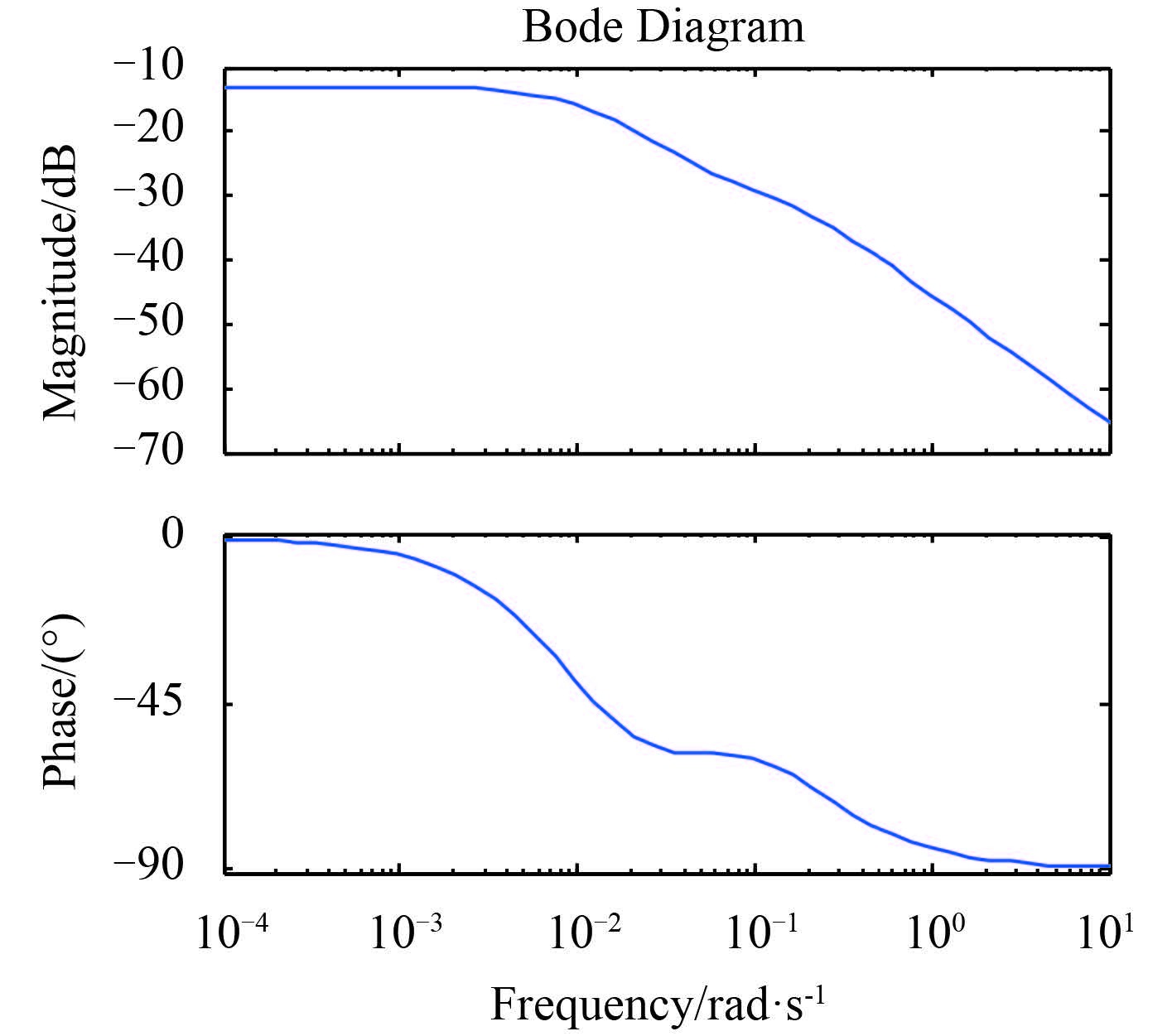图 1 航速 15 kn 下艏摇运动的 Bode 图 Fig. 1 The yawing Bode diagram for velocity of 15 knots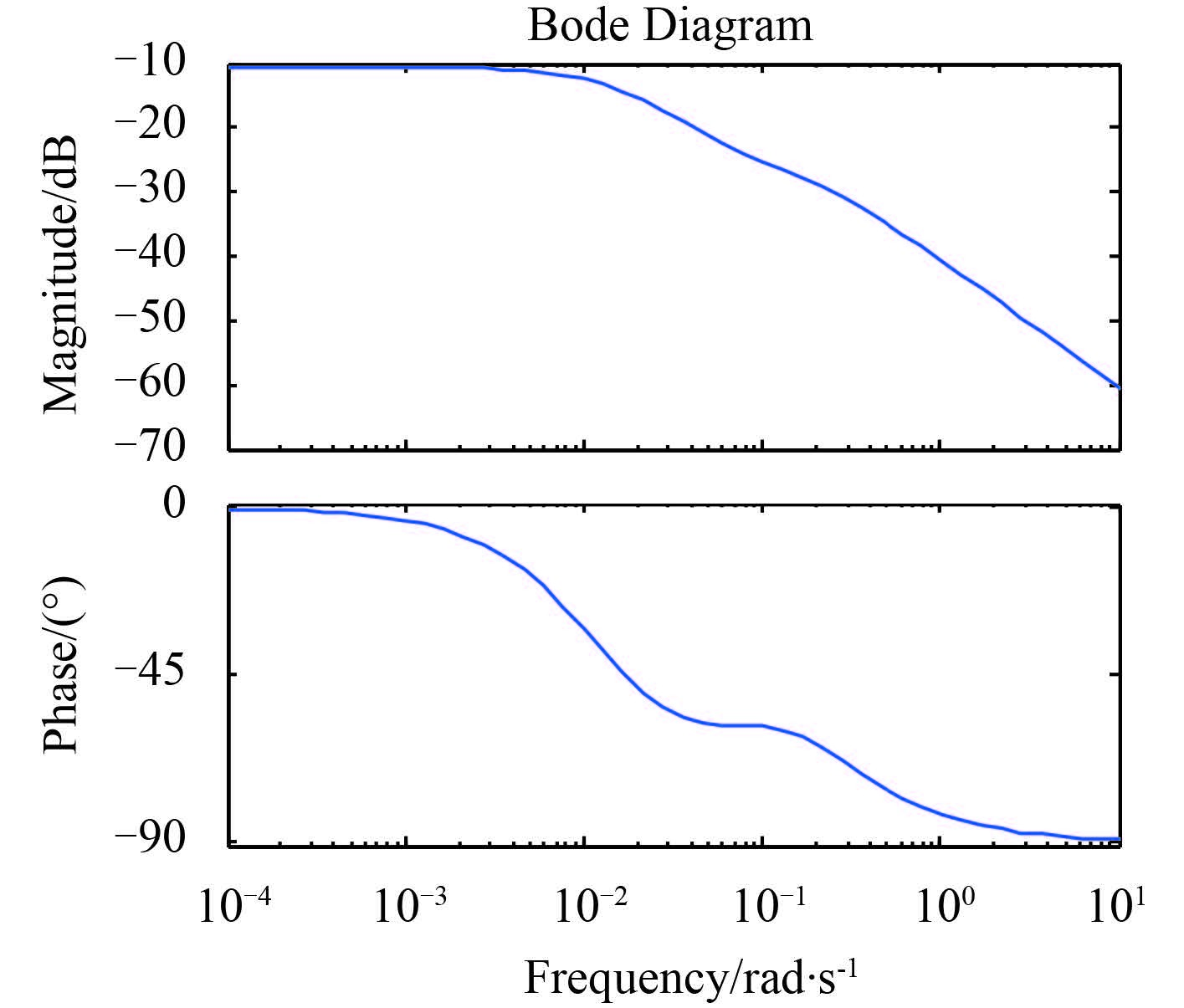图 2 航速 24 kn 下艏摇运动的 Bode 图 Fig. 2 The yawing Bode diagram for velocity of 24 knots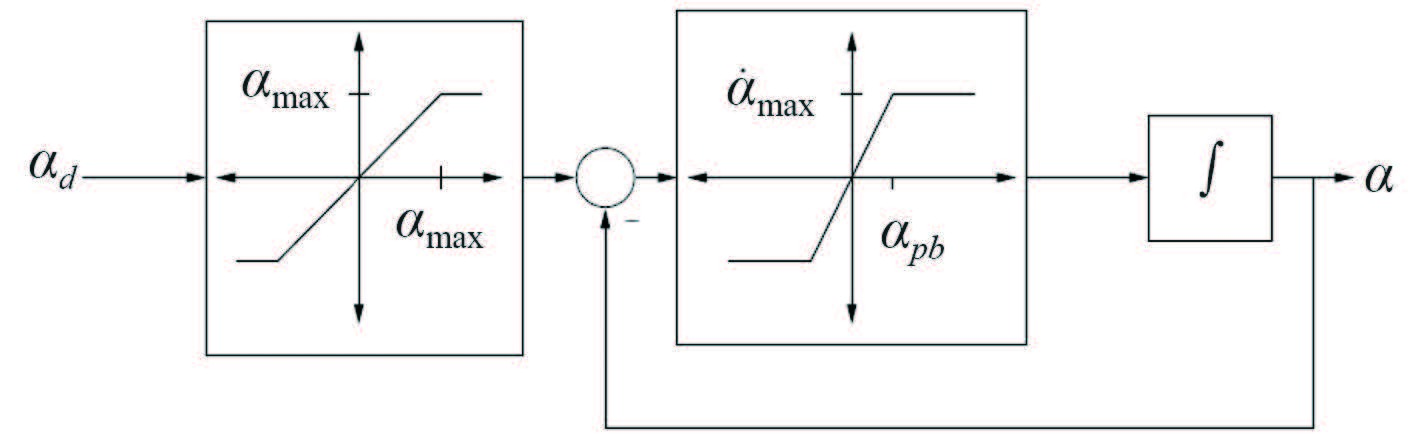图 3 舵液压执行机构模型简化方框图 Fig. 3 The simplified block diagram of rudder hydraulic actuator

 $\frac{\alpha }{{{\alpha _d}}} = \frac{1}{{s + 1}} ,$ (4)

 ${N_{\omega d}} = \frac{1}{2}{\rho _a}g{({V_a} + {V_T})^2}L{C_{n\omega }}({\mu _{\omega d}}){A_L} ,$ (5)

 $\begin{array}{l} N = \displaystyle \sum\limits_{i = 1}^n {{k_1}{R_1}} \left[{\frac{{B_m^2\sin {R_2}({R_3}\cos {R_3} - \sin {R_3})}}{{R_3^2}} - } \right.\\ \left. \frac{{{L_2}\sin {R_3}({R_2}\cos {R_2} - \sin {R_2})}}{{R_2^2}}} \right]{\varsigma _{ai}}\cos ({\omega _{ei}}t + {\varepsilon _{ni}}). \end{array}$ (6)

 ${R_1} =\displaystyle \frac{{\rho g(1 - {e^{ - {k_1}{d_m}}})}}{{{k_1}}},$
 ${R_2} = \displaystyle \frac{{{k_1}L}}{2}\cos {\mu _e},$
 ${R_3} = \displaystyle \frac{{{k_1}{B_m}}}{2}\sin {\mu _e}.$

 ${N_{cd}} = \frac{1}{2}\rho gV_{cd}^2L{C_{nc}}({\mu _{cd}}){A_{Ls}}.$ (7)

2 分数阶 PID 控制器设计方法

 $_aD_t^\alpha = \left\{ {\matrix{ {{{{{\rm{d}}^\alpha }} \over {{\rm{d}}{t^\alpha }}},} & {Re(\alpha ) > 0,} \cr {1,} & {Re(\alpha ) = 0,} \cr {\int\limits_a^t {{{({\rm{d}}\tau )}^{ - \alpha }}} ,} & {Re(\alpha ).} \cr } } \right.$ (8)

 $_{{t_0}}^CD_t^\alpha f(t){ = _{{t_0}}}D_t^{ - (n - \alpha )}[\frac{{{{\rm d}^n}}}{{{\rm d}{t^n}}}f(t)],$ (9)

 $L{\{ _0}D_t^pf(t);s\} = {s^p}F(s) - \sum\limits_{k = 0}^{n - 1} {{s^k}{{{[_0}D_t^{p - k - 1}f(t)]}_{t = 0}}} ,$ (10)

 $u(t) = {K_p}e(t) + \sum\limits_{i = 1}^k {{K_{Di}}D_{ti}^\mu e(t) + \sum\limits_{j = 1}^n {{K_{Ij}}D_{tj}^{ - \lambda }e(t)} } ,$ (11)

 ${G_c}(s) = {K_p} + \sum\limits_{i = 1}^k {{K_{Di}}{s^\mu } + \sum\limits_{j = 1}^n {{K_{Ij}}{s^{ - \lambda }}} } .$ (12)
3 分数阶 PID 控制器设计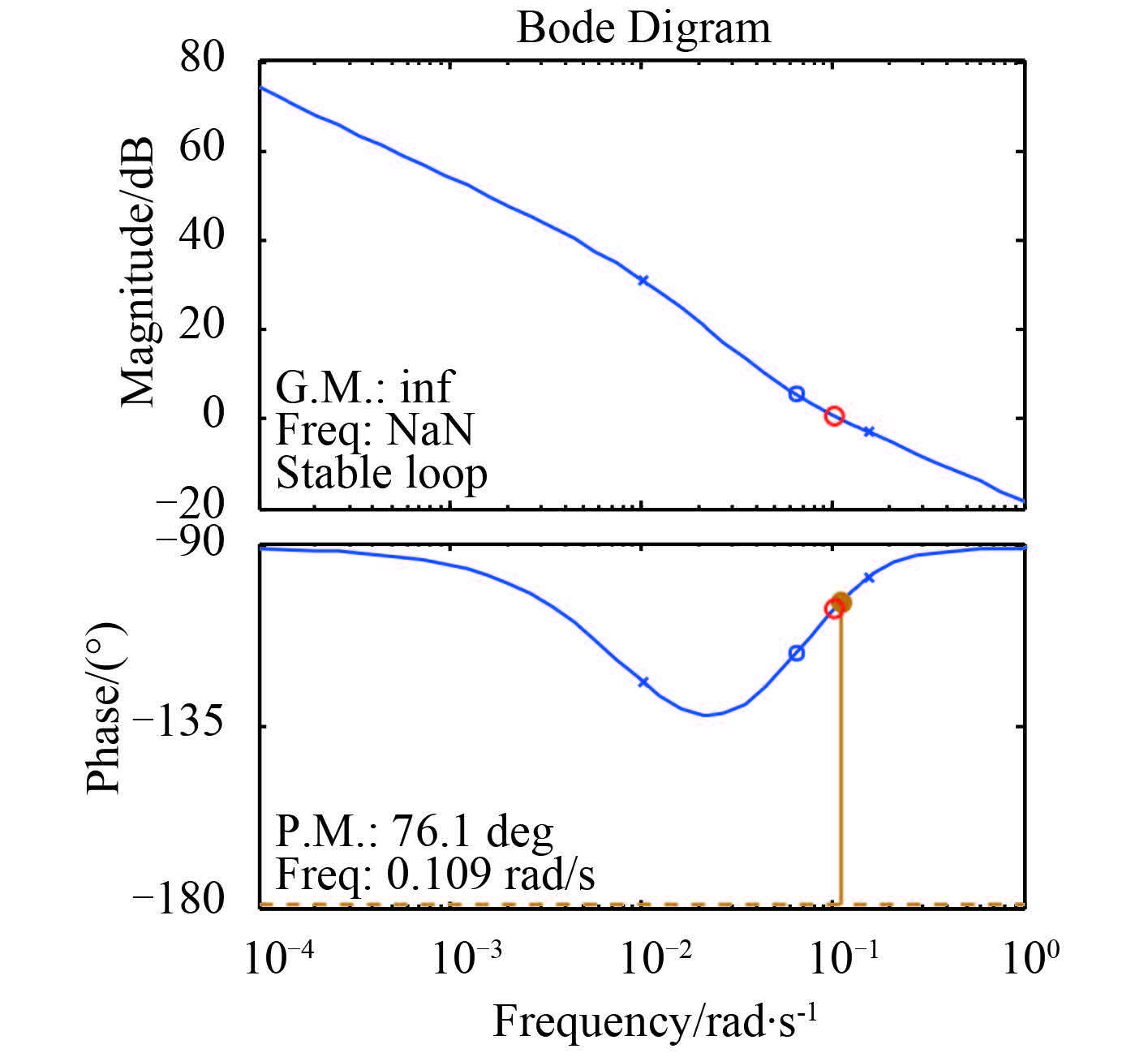图 4 航速 15 kn 下闭环系统 Bode 图 Fig. 4 The Bode diagram of closed loop system for velocity of 15 knots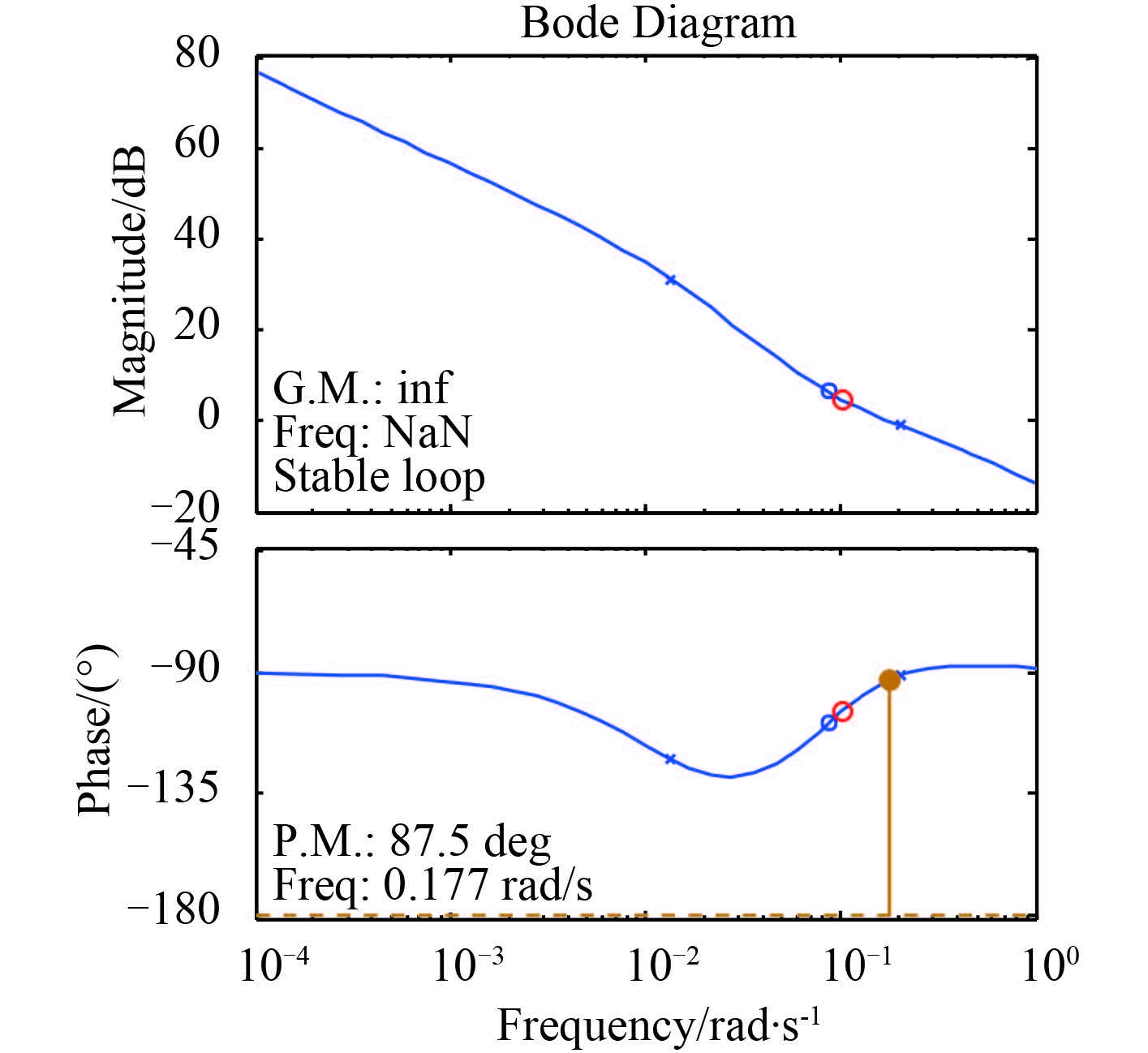图 5 航速 24 kn 下艏摇运动的 Bode 图 Fig. 5 The Bode diagram of closed loop system for velocity of 24 knots

4 仿真试验与数据分析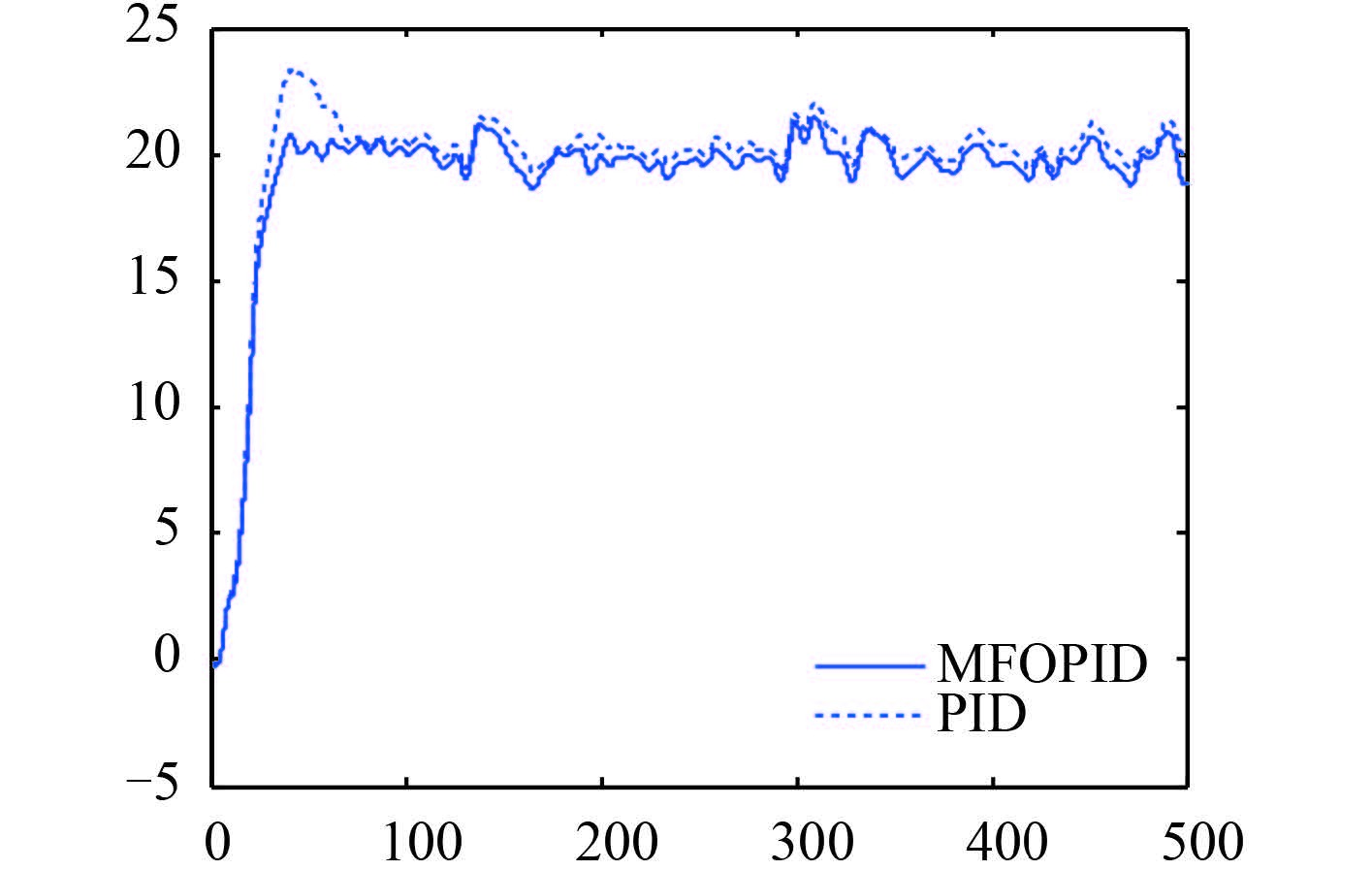图 6 航速 15 kn 下航向保持回路仿真响应曲线图 Fig. 6 The simulating response curves of heading-hold loop for velocity of 15 knots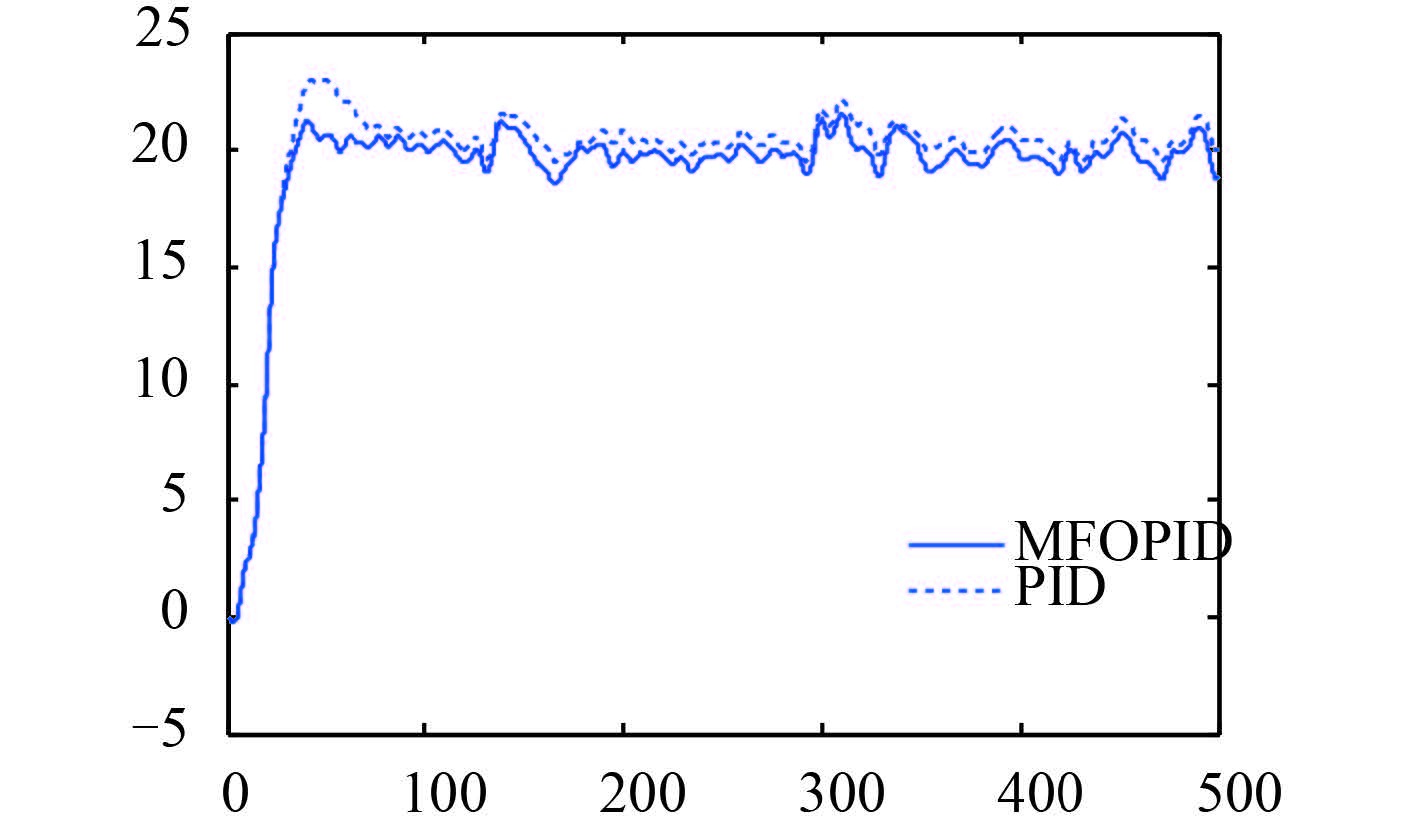图 7 航速 24 kn 下航向保持回路仿真响应曲线图 Fig. 7 The simulating response curves of heading-hold loop for velocity of 24 knots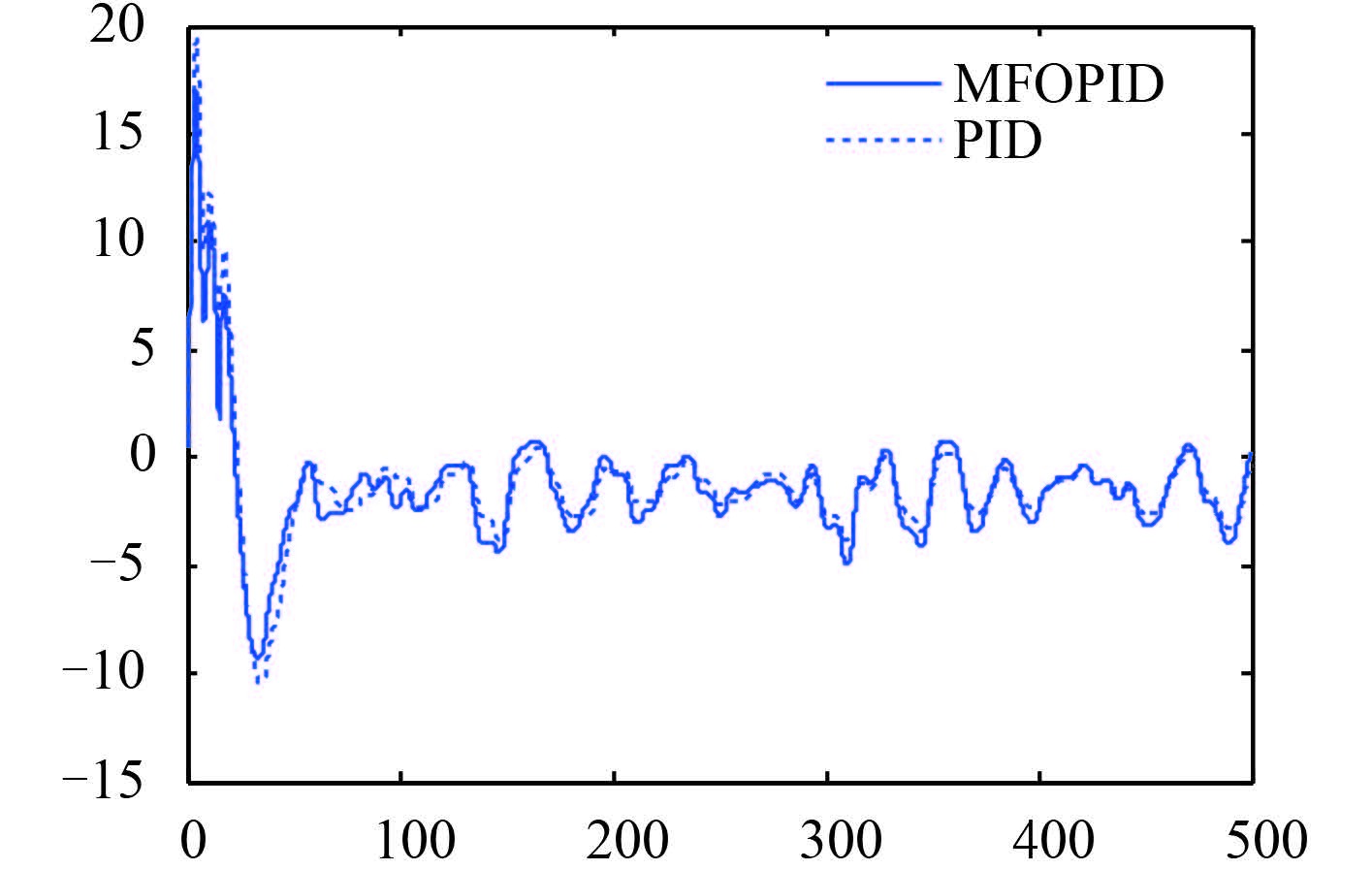图 8 航速 15 kn 下舵角输入曲线图 Fig. 8 The rudder input curves for velocity of 15 knots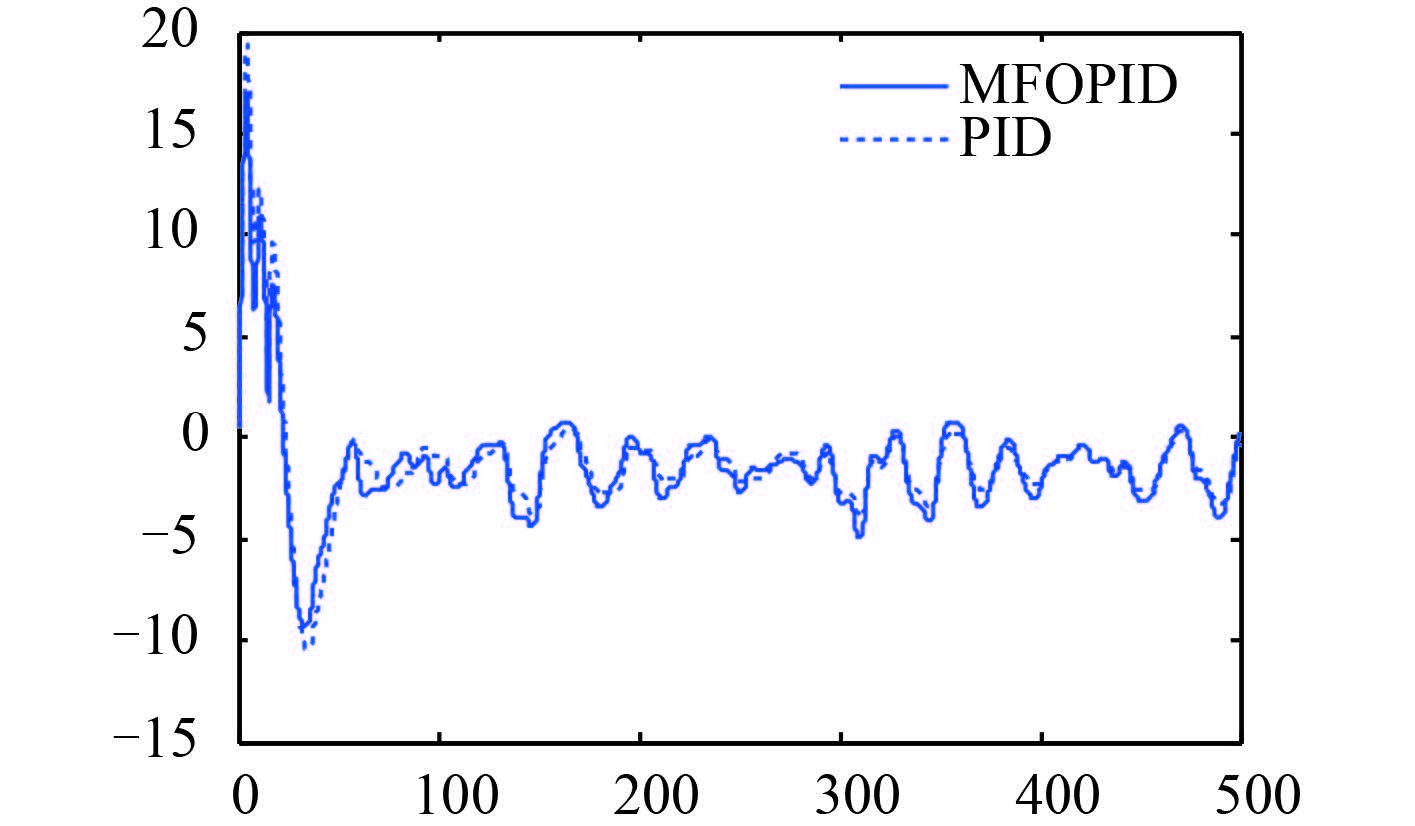图 9 航速 24 kn 下舵角输入曲线图 Fig. 9 The rudder input curves for velocity of 24 knots
5 结语

  范尚雍. 船舶操纵性[M]. 北京: 国防工业出版社, 1988 : 30 -31.  吴金波, 李天奇. 基于交叉耦合的浮筏举升系统模糊PI同步控制[J]. 舰船科学技术 , 2015, 37 (12) :33–37. WU Jin-bo, LI Tian-qi. Synchronized fuzzy PI control for raft lifting system based on cross-coupling[J]. Ship Science and Technology , 2015, 37 (12) :33–37.  PEREZ T. Ship motion control:Course keeping and roll stabil-isation using rudder and fins[M]. London: Springer, 2005 : 107 -109.  金鸿章, 李国斌. 船舶特种装置控制系统[M]. 北京: 国防工业出版社, 1995 : 1 -5.  CHISLETT M S, STR.EM-TEJSEN J. Planar motion mechanism tests and full-scale steering and maneuvering predictions for a mariner class vessel, technical report Hy-6[M]. Lyn-gby, Denmark: Hydro- and Aerodynamics Laboratory, 1965 : 1 -41.  王春阳, 李明秋, 姜淑华, 等. 分数阶控制系统设计[M]. 北京: 国防工业出版社, 2014 : 5 -15.  DORCAK L. Numerical models for the simulation of the fractional-order control systems[R]. UEF-04-94, Kosice, 1994:arXiv:math/0204108.  PODLUBNY I. Fractional differential equations[M]. New York: Academic Press, 1998 : 243 -259.  刘胜. 现代船舶控制工程[M]. 北京: 科学出版社, 2010 : 34 -37.  曾庆山, 曹广益, 王振滨. 分数阶控制器的仿真研究[J]. 系统仿真学报 , 2004, 16 (3) :465–469. ZENG Qing-shan, CAO Guang-yi, WANG Zhen-bin. Simulation research on fractional-order controller[J]. Journal of System Simulation , 2004, 16 (3) :465–469.  金永顺.面向鲁棒运动控制系统的分数阶PID控制器设计、自整定及实验研究[D]. 长沙:湖南大学, 2011:1-7. JIN Yong-shun. Fractional order PID controller synthesis, autotuning and experiment studies for robust motion control systems[D]. Changsha:Hunan University, 2011:1-7.  卢京潮. 自动控制原理[M]. 西安: 西北工业大学出版社, 2009 : 135 -150.